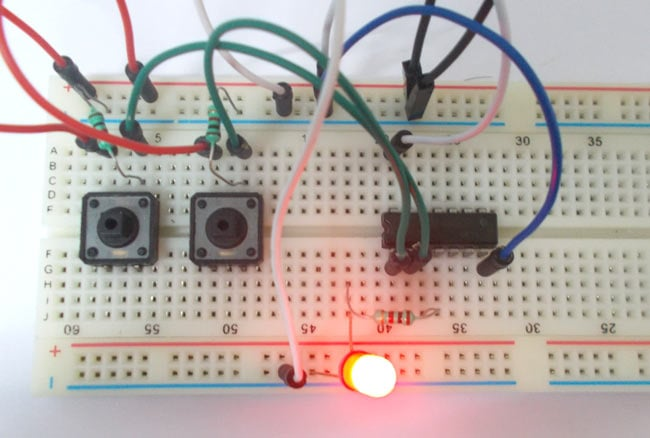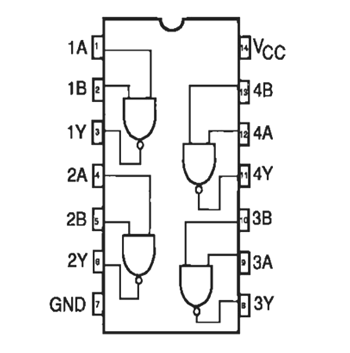# NAND Gate

Published  August 25, 2015   0NAND gate is a digital logic gate, designed for arithmetic and logical operations, every electronic student must have studied this gate is his/her career. This gate is mainly used in applications where there is a need for mathematical calculations. So calculators, computers and many digital applications use this gate.

Here we are going to use 74LS00 IC for demonstration, this chip has 4 NAND gates in it. These four gate are connected internally as shown in below figure.These gates have limitations for working voltage and input logic frequency. When these limitations are not considered the chip may damage permanently, so one should pay attention while selecting the  logic gates.

### Components Required

• Power supply (5v)
• 1K and 220Ω resistors
• 74LS00 QUAD NAND GATE IC
• 1 LED
• Buttons
• 100nF capacitor
• Connecting wires

### Circuit Diagram and Working Explanation

The truth table of NAND gate is show in figure.

 A B Y L L H L H H H L H H H L

As in truth table the output of a NAND gate should be low only if both the gate inputs are high.  In any other case the output should be high. So if any one or both inputs are low the output of NAND gate will be high.

In this NAND gate circuit diagram we are going to pull down both input of a gate to ground through a 1KΩ resistor. And then the inputs are connected to power through a button.So when the button is pressed the corresponding pin of gate goes high. So with two buttons we can realize the truth table of NAND gate. When one of button is pressed one input of gate will be high and other will be low at this time the output should be high.

So by truth table, the LED should be off only when both buttons are pressed. If any or either buttons are released the LED should be ON.

The capacitor is for neutralizing the bouncing effect of the button. In the absence of capacitor, the counter may count the events occurring wrongly. These pull down resistors are necessary as the chosen CHIP is a positive edge triggering one. If the resistors are ignored the circuit might generate unpredictable results. (Also check: NOT Gate Circuit)

Video